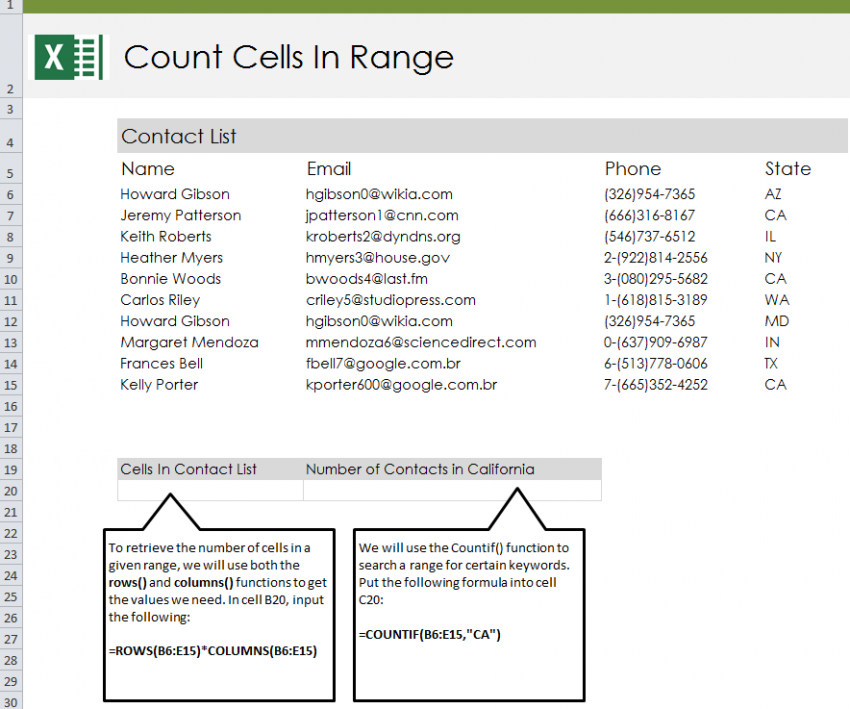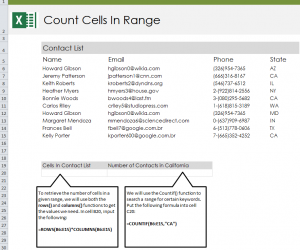# Count Cells with Text in Excel13
AprFollow our guide and learn how to count cells with text in Excel spreadsheets. Excel has a formula that you can enter that will search a range of cells and return to you the number of cells within that range that contain any sort of text. Along with that feature, there is the COUNTIF formula which allows you to search cells in a range containing certain text entries. It will then return to you the number of cells that contain that specific text.

Open your downloaded example and you will see a contact list containing first and last names, email addresses, phone numbers, and states that indicate where the contact lives. The goal of this example is to do two things: have the formula count the total number of cells with text in them, and count the number of cells with certain text.

Start by selecting cell B20 and entering this formula:

=ROWS(B6:E15)*COLUMNS(B6:E15)

The result in B20 should be the number 40.

The formula you entered tells Excel to search the columns and rows between cell B6 and cell E15. You can change the cells to get different results. Experiment with the cells to view numbers for different ranges.

Next, try out the example to learn how to use the COUNTIF formula, which will teach you how to isolate text and count how many cells contain that text. in cell C20, enter this formula:

=COUNTIF(B6:E15,”CA”)

This formula tells Excel to search all the cells from B6 to E15 and find the cells that contain “CA”. The result will be the number 3. Try changing the word “CA” to something else to practice isolating text in ranges.